#### Chapter 10 Congruent Triangles R.D. Sharma Solutions for Class 9th Exercise 10.1

Exercise 10.1

1. In Fig. 10.22, the sides BA and CA have been produced such that BA = AD and CA = AE. Prove that segment DE || BC.Solution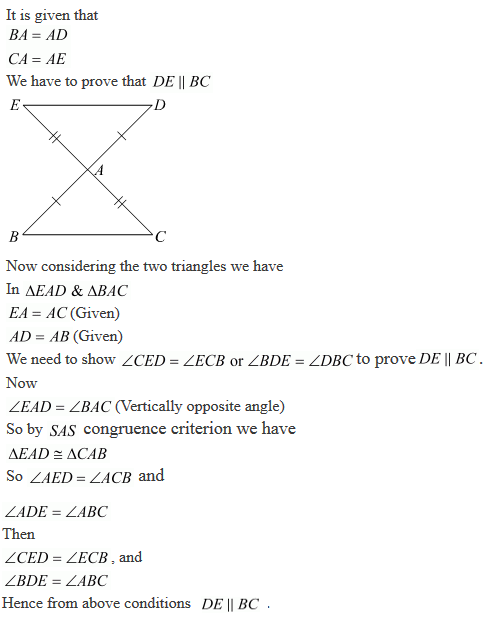2. In a ΔPQR, if PQ = QR and L, M and N are the mid-points of the sides PQ, OR, and RP respectively. Prove that LN = MN.

Solution3. In Fig. 10.23, PQRS is a square and SRT is an equilateral triangle. Prove that
(i) PT = QT
(ii) ∠TQR = 15°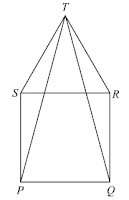Solution(ii)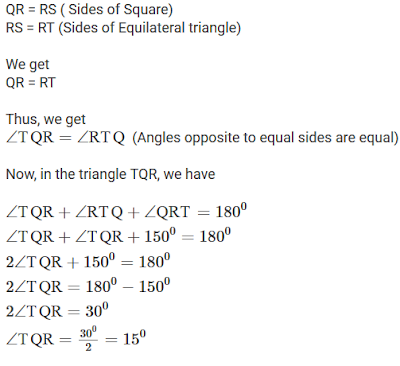4. Prove that the medians of an equilateral triangle are equal.

Solution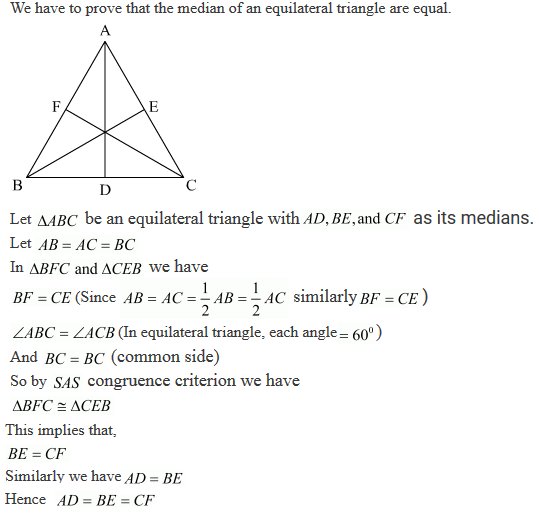5. In a Δ ABC, if ∠A = 120° and AB = AC. Find ∠B and ∠C.

Solution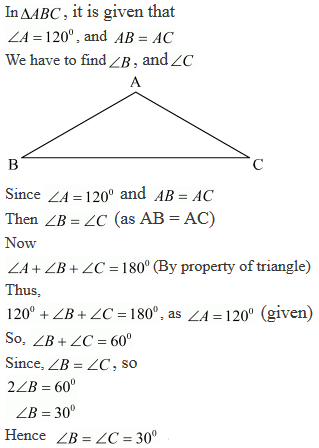6. In a ΔABC, if AB = AC and ∠B = 70°, find ∠A.

Solution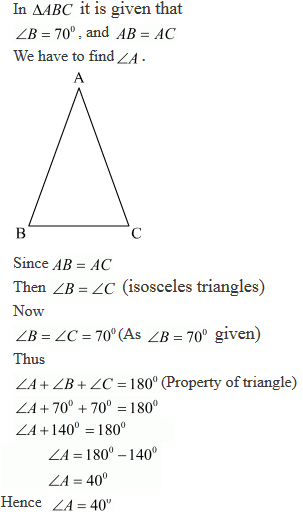7. The vertical angle of an isosceles triangle is 100°. Find its base angles.

Solution8. In Fig. 10.24, AB = AC and ∠ACD = 105°, find ∠BAC.Solution9. Find the measure of each exterior angle of an equilateral triangle.

Solution10. It is given that the base of an isosceles triangle is produced on both sides.

Solution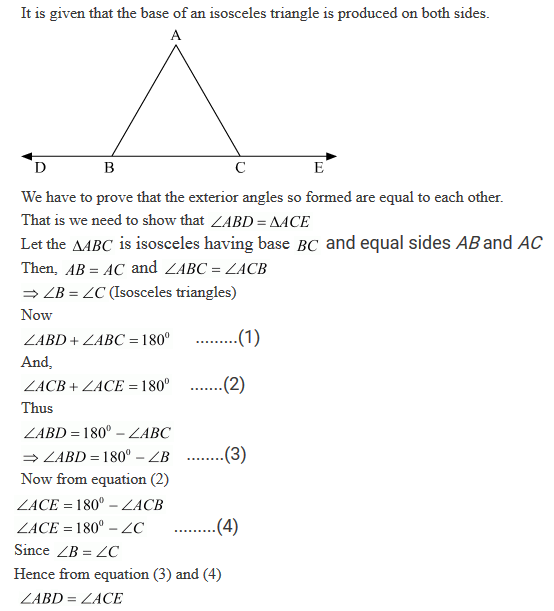11. In Fig. 10.25, AB = AC and DB = DC, find the ratio ∠ABD : ∠ACD.Solution12. Determine the measure of each of the equal angles of a right-angled isosceles triangle.
OR ABC is a right-angled triangle in which ∠A = 90° and AB = AC. Find ∠B and ∠C.

Solution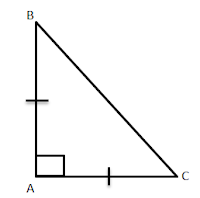It is given that,
ABC is right angled triangle where,
∠A = 90°
AB = AC
We have to find ∠B and ∠C.
Since, AB = AC
∠B = ∠C  (Isoceles triangle)
Now,
∠A +∠B+∠C = 180  (Property of triangle)
⇒ 90 + 2∠B = 180  (∠B = ∠C)
⇒ 2∠B = 180 - 90
⇒ ∠B = 45
So, ∠B = ∠C = 45
Hence,
∠B = 45° and ∠C = 45°

13. AB is a line segment. P and Q are points on opposite sides of AB such that each of them is equidistant from the points A and B (See Fig. 10.26). Show that the line PQ is perpendicular bisector of AB.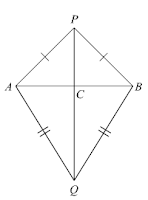Solution